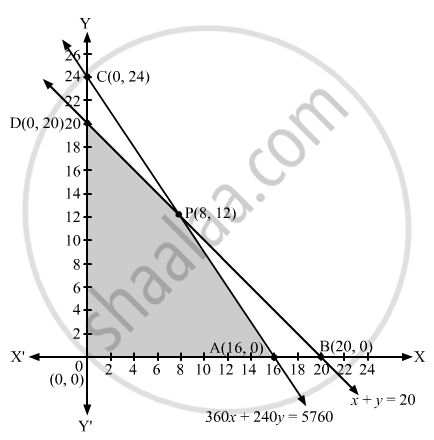# A dealer in rural area wishes to purchase a number of sewing machines. He has only Rs 5,760 to invest and has space for at most 20 items for storage. - Mathematics

A dealer in rural area wishes to purchase a number of sewing machines. He has only Rs 5,760 to invest and has space for at most 20 items for storage. An electronic sewing machine cost him Rs 360 and a manually operated sewing machine Rs 240. He can sell an electronic sewing machine at a profit of Rs 22 and a manually operated sewing machine at a profit of Rs 18. Assuming that he can sell all the items that he can buy, how should he invest his money in order to maximize his profit? Make it as a LPP and solve it graphically.

#### Solution

Suppose that a dealer buys x electronic sewing machines and y manually operated sewing machines.
The dealer has space for at most 20 items.
∴ x y ≤ 20

Now, an electronic sewing machine costs Rs 360 and a manually operated sewing machine costs Rs 240.
Therefore, the total cost of x electronic sewing machines and y manually operated sewing machines is Rs (360x + 240y).
But the dealer has only Rs 5760 to invest.
∴ 360x + 240y ≤ 5760

The dealer can sell all the items that he can buy. Since, the profit on an electronic sewing machine is Rs 22 and that on a manually operated sewing machine is of Rs 18, the total profit is of Rs (22x + 18y).

Let Z denote the total profit.
Then Z = 22x + 18y
Clearly, x, y ≥ 0
Thus, the mathematical formulation of the given problem is
Maximize Z = 22x + 18y

Subject to the constraints:
x y ≤ 20                             ...(i)
360x + 240y ≤ 5760              ...(ii)
and x, y ≥ 0                          ...(iii)
Let us draw the graph of constraints (i), (ii) and (iii).
APDO, as shown in the figure below, is the feasible region (shaded) determined by the constraints (i), (ii) and (iii).The corner points of the feasible region are A(16, 0), P(8, 12), D(0, 20) and O(0, 0).
Let us evaluate Z = 22x + 18y at these corner points.

 Corner point Z = 22x + 18y (16, 0) 352 (8, 12) 392 (0, 20) 360 (0, 0) 0

We see that the point (8, 12) is giving the maximum value of Z.
Hence, the dealer should purchase 8 electronic sewing machines and 12 manually operated sewing machines to obtain the maximum profit under the given conditions.

Concept: Graphical Method of Solving Linear Programming Problems
Is there an error in this question or solution?
2013-2014 (March) Delhi Set 1

Share